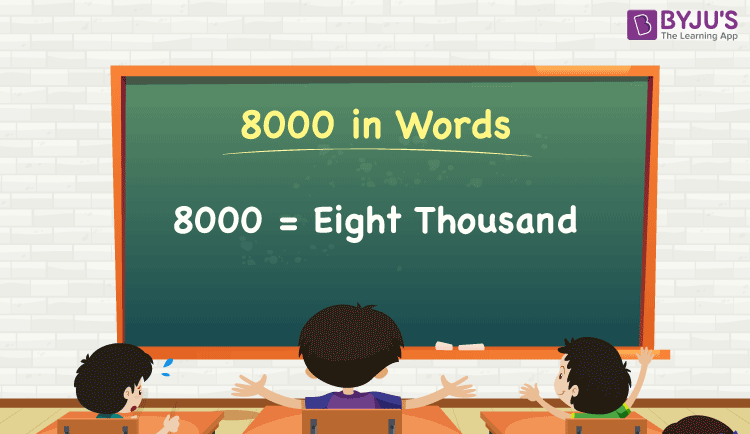# 8000 in Words

8000 in words can be written as Eight thousand. If the number 8000 represents a certain amount of money/the price of a particular thing/total cost of goods, etc., then we express it as Eight thousand rupees. Also, note that 8000 is a cardinal number since it denotes a specific quantity.

 8000 in words: Eight thousand Eight thousand in numerical form: 8000

8000 in English Words

We generally write numbers in words using the English alphabet. Thus, we can read 8000 using the words in English as “Eight thousand”.## How to Write 8000 in Words?

We can convert 8000 to words using a place value chart. This can be done as follows.

The number 8000 has 4 digits, so let’s make a chart that shows the place value up to 4 digits.

 Thousands Hundreds Tens Ones 8 0 0 0

In the above place value chart, we can observe that the digits in ones, tens, and hundreds are 0s. Hence, we can consider the number at the thousands place, and by combining these terms, we get Eight thousand since 8 × 1000 = 8000.

Alternative method:

We can write the expanded form of 8000 as given below:

8 × Thousand + 0 × Hundred + 0 × Ten + 0 × One

= 8 × 1000 + 0 × 100 + 0 × 10 + 0 × 1

= 8000

= Eight thousand

Interesting way of writing 8000 in words:

8 = Eight

80 = Eighty

800 = Eight hundred

8000 = Eight thousand

### Facts About the Number 8000

8000 is a natural number which is the successor of 7999 and the predecessor of 8001.

8000 in words – Eight thousand

Is 8000 an odd number? – No

Is 8000 an even number? – Yes

Is 8000 a prime number? – No

Is 8000 a composite number? – Yes

Is 8000 a perfect square number? – No

Is 8000 a perfect cube number? – Yes

What is the cube root of 8000? – 20

## Frequently Asked Questions on 8000 in Words

Q1

### How do you write 8000 in English?

The spelling of 8000 in English words is Eight thousand, which means 8000 in words can be expressed as Eight thousand.

Q2

### How do you write 8000 in word form?

The word form of the number 8000 is eight thousand.

Q3

### How do you write 8000 in words on a cheque?

On a cheque, we generally write 8000 in words as Eight thousand rupees only.

Q4

### What is the sum of Rs. 7000 and Rs. 1000in words?

The sum of Rs. 7000 and Rs. 1000 is Rs. 8000, i.e. eight thousand rupees.